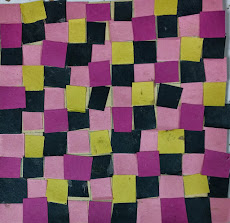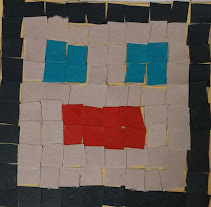# Coloring Tiles - Decimal Designs

72 teachers like this lesson
Print Lesson

## Objective

SWBAT identify tenths and hundredths and represent fractions with denominators of 10 and 100 as a decimal.

#### Big Idea

In this lesson, students combine art and math to understand decimal notation for fractions, and compare decimal fractions.

## Concept development

40 minutes

In this hands on lesson, students participate in a tactile activity as a way to grasp the connections among fractions and decimals.  In this introductory lesson, students use what they know about fractions to begin their exploration of decimals. By connecting fractions to decimals, students will build a deeper understanding of decimals. They explore decimals using Base Ten Blocks and 10x10 grids.

Note: In preparing for the lesson, I used a grid of 100 squares on tan graph paper so that students could clearly visualize and determine the decimal form (0.01 for each square) for the amount of each color  used. Students could then calculate their fraction and decimal equivalents. Each student was required to use at least three colors of squares. Squares left blank could be counted as white or tan. I asked students to choose from a total of six different colors but ultimately left the design of the artwork to them.

I instruct students to glue squares onto a 10 x 10 grid to create a mosaic design. They must use between 3 and 6 colors to fill their grids.  I use www.timeme.com and set the timer for 30 minutes for students to create and glue their designs. This helps keep chatting to a minimum and students focused on the task.

After the 30 minutes, I then direct students to look at their design and calculate the fraction of each color they used.  This is similar to a previous lesson, so my students should not struggle with this at all.

Students record the fraction for each color they used on the back of their mosaic grid. Next, I tell students that there are many ways to represent numbers.  I display a 10 x 10 grid under the document camera and shade in 10 squares.  I ask the students how many squares make the whole. (100)  Then I ask the students what part of the whole is shaded. (10/100)  I tell students that another name for this number is one tenth or 0.10.  I tell students that this second number is a decimal and invite them to list where they have seen decimals before.

Next, I show students different grids and we practice together naming the fraction and the decimal together.  (i.e 62/100  = 0.62 = sixty two hundredths.)  I show students that 0.62 is 6/10 + 2/100 = 62/100

Finding the number of a certain color of square is one way to give students an opportunity to think about pairs that make 100. As students make their decimal designs on the 10 x 10 grid, I ask them if they have more of particular colors. If they have more of a color, I ask them to count the number of squares that are not that color and subtract that number from 100 (i.e. think about what number added to the number of unshaded squares would equal 100). This is a great opportunity to review numbers that add up to 100 and practice mental math and for students to explain how they know how many squares are shaded.

The following are two examples of fraction mosaics my students created.